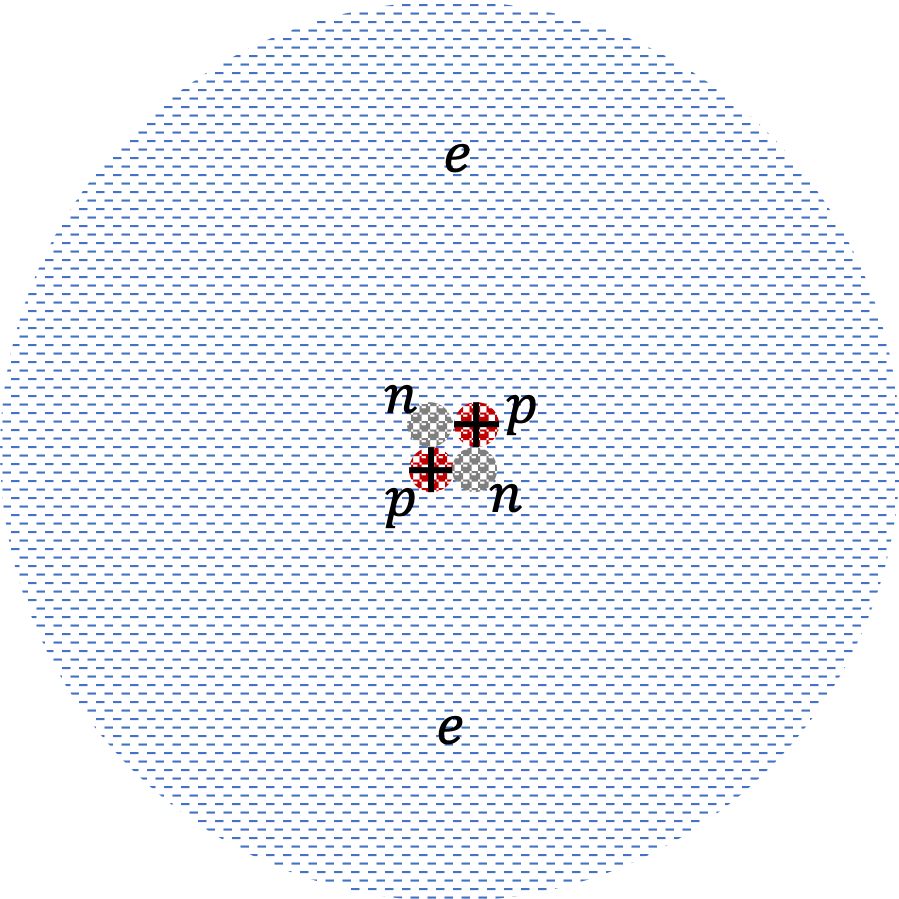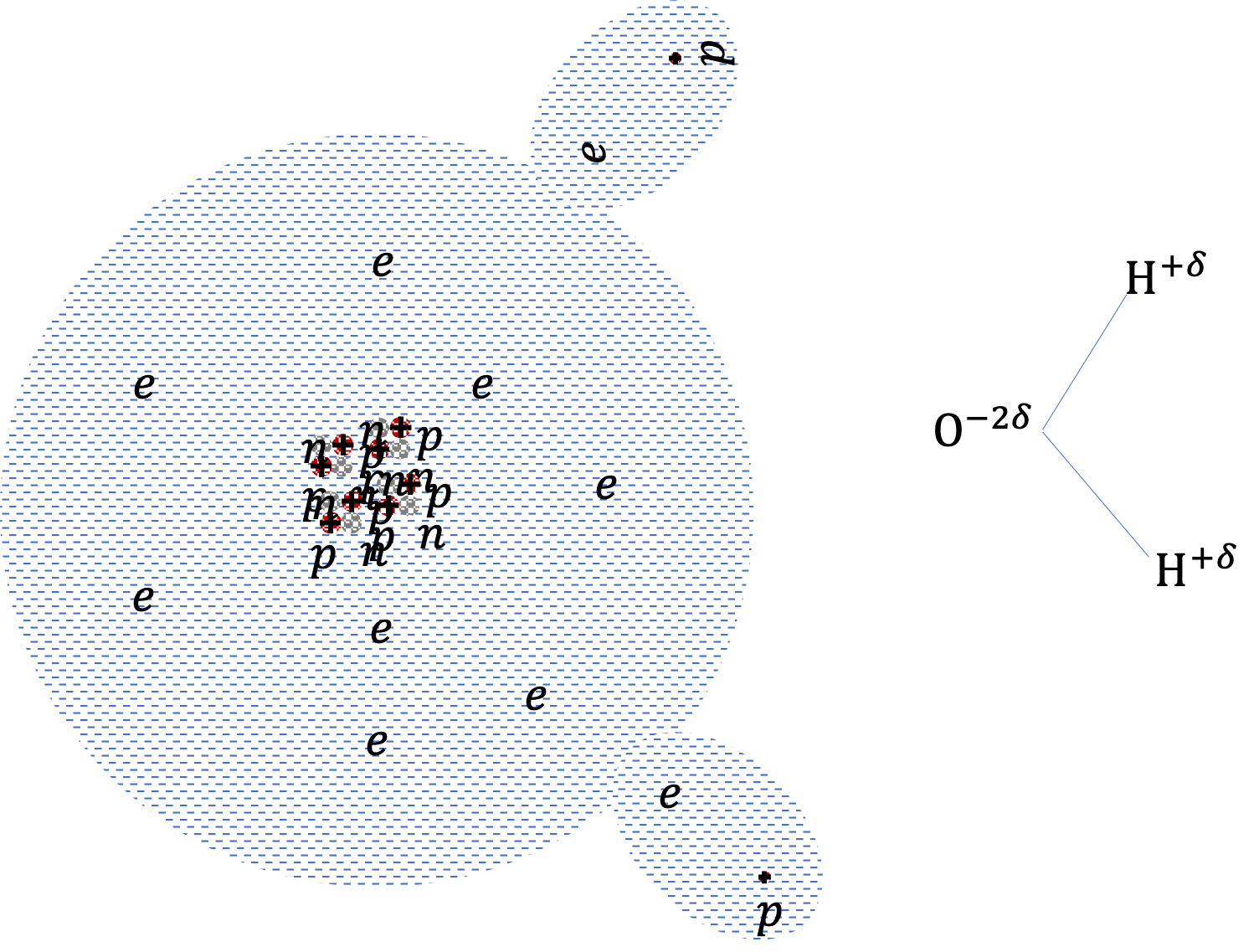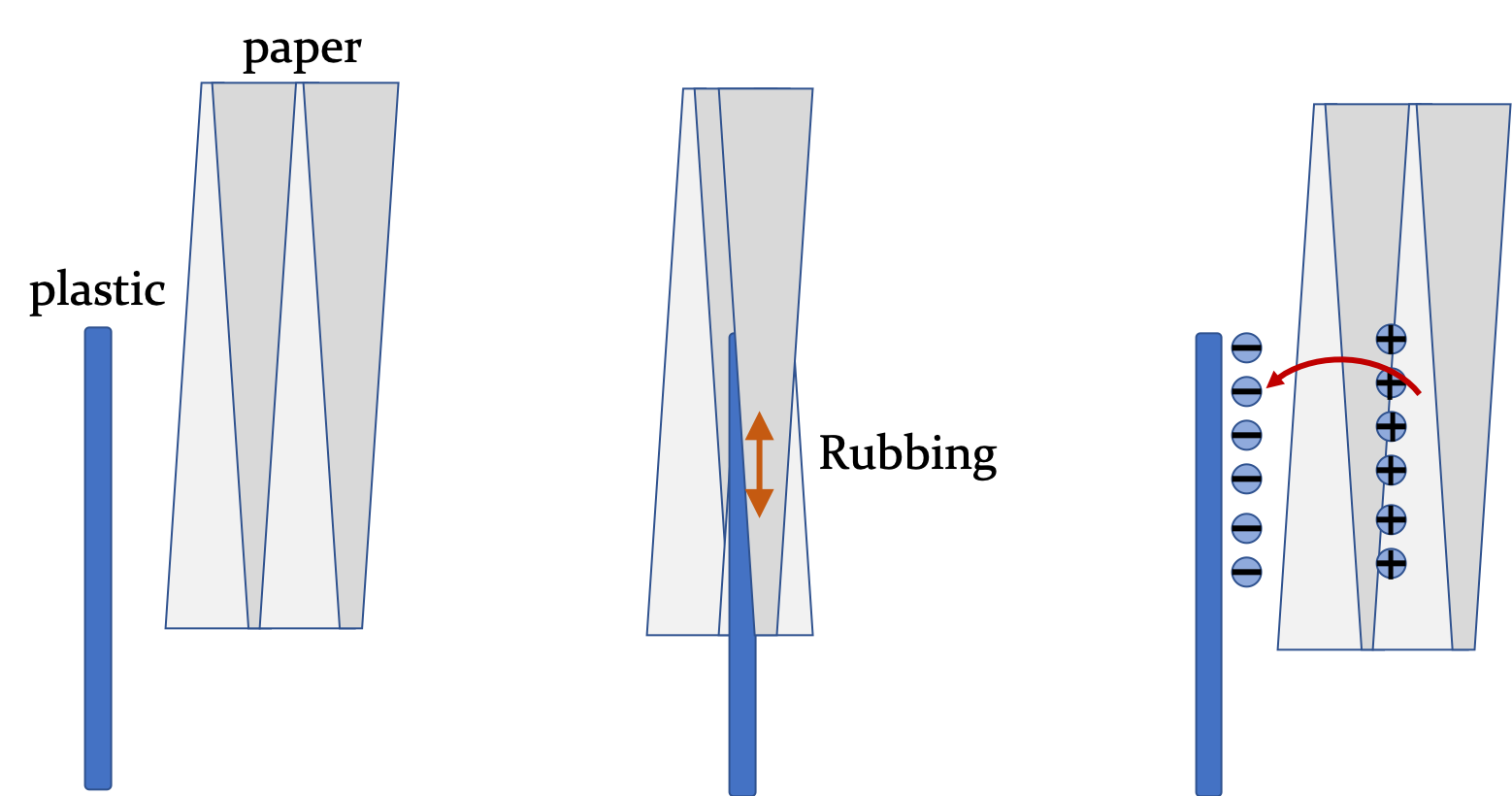## Section28.2Microscopic Origin of Electricity

If you rub a neutral plastic rod with a neutral paper towel, you will find that the plastic rod and the paper towel become charged. What is going on inside these materials for them to develop excess charges?

In order to understand the electrification process, we must look at the atomic level. Atoms have an equal number of electrons and protons. It is a fundamental fact of nature that electrons and protons have exactly equal charges of opposite types.

Traditionally, an electron's charge, denoted as $-e\text{,}$ has been given a negative sign, where $e$ is called electronic charge. The value of $e$ in Coulomb, the SI unit named after the French physicist Charles Augustin de Coulomb (1736-1806), who conducted valuable experiments with static electricity.

\begin{equation} e=\left(1.60217733 \pm 0.00000049 \right)\times 10^{-19}\ \textrm{C}.\tag{28.2.1} \end{equation}

The charge of an electron is one of the most precisely measured quantities in physics with the following experimental value. All objects with the same type of charges as that of an electron are called negatively charged. A proton then has a positive charge $+e\text{,}$ and all objects whose charges are of the same type as that of a proton are called positively charged.

In atoms, electrons are distributed around a tiny nucleus, which contains all the protons as illustrated in the figure on the right for a Helium atom. Nucleus also contains neutral particles called neutrons. Atoms of different chemical elements differ in their abilities to hold onto their own electrons and to attract charges from outside the atom.Some atoms, such as atoms of sodium, readily lose an entire electron and become positively charged ions, while others gain an entire electron, such as atoms of chlorine, and become negatively charged ions. In Chemistry, we designate these ions with the symbol of the element and the amount of charge in units of $e\text{.}$ For instance, a sodium atom that has lost an electron is designated as $\text{Na}^{+}$ and a chlorine atom that has gained an electron is designated as $\text{Cl}^{-}\text{.}$ These ions are found in the common salt.

Metals behave as if some electrons of each atom, called the conduction electrons, are not bound to any one particular atom but free to roam about in the entire metal. In non-metals, also called insulators, electrons are localized near each atom.

When two atoms of different elements are next to each other a polarization of charges results with one atom developing a negative charge and the other developing the same amount of positive charge. For instance, in the water molecule, the oxygen atom is negatively charged while the two hydrogen atoms are positively charged. Such substances are called $polar\text{.}$ Much of useful properties of water can be traced to the electrical polarization of its molecules.Figure 28.2.1. Illustration of electronic structure of a water molecule. The oxygen atom pulls electrons closer to itself, which polarizes the molecule. Unlike full ionization, hydrogen does not lose its electron fully. The charge on electron is denoted by $-\delta\text{,}$ which is a fraction of electron's charge.

The competition for electrons is also at play when two dissimilar materials are rubbed or pressed against each other. In the case of a plastic rod rubbed with a paper towel as shown in illustration Figure 28.2.2, the electrons move from paper towel to the plastic rod making the rod negatively charged since the molecules in the plastic rod have greater attraction for the electrons than the molecules in the paper. After rubbing, the towel has a fewer electrons than what is needed to balance the charges on its protons, and therefore would be positively charged on the whole. When you rub two dissimilar materials, you do not create charges, but rather, you transfer electrons from one material to the other. The net charge is still the same after the process as before.Figure 28.2.2. Rubbing of a plastic rod by paper towel transfers electrons from paper to plastic.